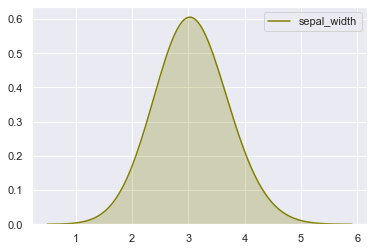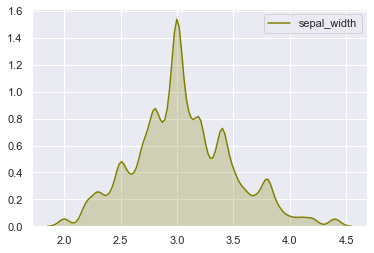# Control the bandwidth of a seaborn density plot

As explained in seaborn documentation, kdeplots display information in a similar way than histograms, though a Gaussian kernel is used to produce a smoothed line corresponding to observations count. Just as you could modify histograms bins size in order to categorize data, you can also change the way a kdeplot is built in order to get a better understanding of the data distribution.

The following density plots have been made using the same data. Only the bandwidth value changes from 0.5 in the first graph to 0.05 on the right. This is controlled using the bw argument of the kdeplot function (seaborn library).

This parameter can be of particular interest when a finer understanding of the distribution is needed. It could highlight bimodal distributions more easily and help us in observing patterns that the Gaussian kernel over-smoothed.

Note that 'bw' parameter is deprecated since seaborn 0.11.0 and 'bw_method' and 'bw_value' are to be used. See scipy.stats.gaussian_kde in scipy.org for further details on bw_method and bw_value.

``````# libraries & dataset
import seaborn as sns
import matplotlib.pyplot as plt
# set a grey background (use sns.set_theme() if seaborn version 0.11.0 or above)
sns.set(style="darkgrid")
df = sns.load_dataset('iris')

# Large bandwidth
sns.kdeplot(df['sepal_width'], shade=True, bw=0.5, color="olive")
plt.show()``````In seaborn 0.11.0 and later versions, you would use sns.kdeplot(df['sepal_width'], shade=True, bw_method=0.05, color='olive')

``````# libraries & dataset
import seaborn as sns
import matplotlib.pyplot as plt
# set a grey background (use sns.set_theme() if seaborn version 0.11.0 or above)
sns.set(style="darkgrid")
df = sns.load_dataset('iris')

# Narrower bandwidth
sns.kdeplot(df['sepal_width'], shade=True, bw=0.05, color='olive') # if using seaborn < 0.11.0
plt.show()``````## Contact & Edit

👋 This document is a work by Yan Holtz. Any feedback is highly encouraged. You can fill an issue on Github, drop me a message onTwitter, or send an email pasting `yan.holtz.data` with `gmail.com`.

This page is just a jupyter notebook, you can edit it here. Please help me making this website better 🙏!